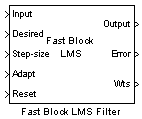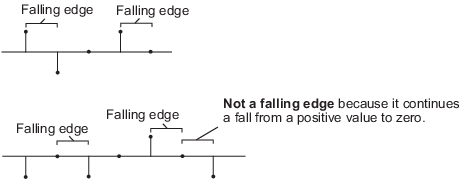# Fast Block LMS Filter

Compute output, error, and weights using LMS adaptive algorithm

## Library

`dspadpt3`

•## Description

The Fast Block LMS Filter block implements an adaptive least mean-square (LMS) filter, where the adaptation of the filter weights occurs once for every block of data samples. The block estimates the filter weights, or coefficients, needed to convert the input signal into the desired signal. Connect the signal you want to filter to the Input port. The input signal can be a scalar or a column vector. Connect the signal you want to model to the Desired port. The desired signal must have the same data type, complexity, and dimensions as the input signal. The Output port outputs the filtered input signal. The Error port outputs the result of subtracting the output signal from the desired signal.

The block calculates the filter weights using the Block LMS Filter equations. For more information, see Block LMS Filter. The Fast Block LMS Filter block implements the convolution operation involved in the calculations of the filtered output, y, and the weight update function in the frequency domain using the FFT algorithm used in the Overlap-Save FFT Filter block. See Overlap-Save FFT Filter (Obsolete) for more information.

Use the Filter length parameter to specify the length of the filter weights vector.

The Block size parameter determines how many samples of the input signal are acquired before the filter weights are updated. The input frame length must be a multiple of the Block size parameter.

The Step-size (mu) parameter corresponds to µ in the equations. You can either specify a step-size using the input port, Step-size, or enter a value in the Block Parameters: Block LMS Filter dialog box.

Use the Leakage factor (0 to 1) parameter to specify the leakage factor, $0<1-\mu \alpha \le 1$, in the leaky LMS algorithm shown below.

`$w\left(k\right)=\left(1-\mu \alpha \right)w\left(k-1\right)-f\left(u\left(n\right),e\left(n\right),\mu \right)$`

Enter the initial filter weights, $w\left(0\right)$, as a vector or a scalar in the Initial value of filter weights text box. When you enter a scalar, the block uses the scalar value to create a vector of filter weights. This vector has length equal to the filter length and all of its values are equal to the scalar value.

When you select the Adapt port check box, an Adapt port appears on the block. When the input to this port is nonzero, the block continuously updates the filter weights. When the input to this port is zero, the filter weights remain at their current values.

When you want to reset the value of the filter weights to their initial values, use the Reset input parameter. The block resets the filter weights whenever a reset event is detected at the Reset port. The reset signal rate must be the same rate as the data signal input.

From the Reset input list, select `None` to disable the Reset port. To enable the Reset port, select one of the following from the Reset input list:

• `Rising edge` — Triggers a reset operation when the Reset input does one of the following:

• Rises from a negative value to a positive value or zero

• Rises from zero to a positive value, where the rise is not a continuation of a rise from a negative value to zero (see the following figure)• `Falling edge` — Triggers a reset operation when the Reset input does one of the following:

• Falls from a positive value to a negative value or zero

• Falls from zero to a negative value, where the fall is not a continuation of a fall from a positive value to zero (see the following figure)• `Either edge` — Triggers a reset operation when the Reset input is a `Rising edge` or `Falling edge` (as described above)

• `Non-zero sample` — Triggers a reset operation at each sample time that the Reset input is not zero

Select the Output filter weights check box to create a Wts port on the block. For each iteration, the block outputs the current updated filter weights from this port.

## Parameters

Filter length

Enter the length of the FIR filter weights vector. The sum of the Block size and the Filter length must be a power of 2.

Block size

Enter the number of samples to acquire before the filter weights are updated. The number of rows in the input must be an integer multiple of the Block size. The sum of the Block size and the Filter length must be a power of 2.

Specify step-size via

Select `Dialog` to enter a value for mu, or select `Input port` to specify mu using the Step-size input port.

Step-size (mu)

Leakage factor (0 to 1)

Enter the leakage factor, $0<1-\mu \alpha \le 1$. Tunable (Simulink).

Initial value of filter weights

Specify the initial values of the FIR filter weights.

Select this check box to enable the Adapt input port.

Reset input

Select this check box to enable the Reset input port.

Output filter weights

Select this check box to export the filter weights from the Wts port.

## References

Hayes, M.H. Statistical Digital Signal Processing and Modeling. New York: John Wiley & Sons, 1996.

## Supported Data Types

PortSupported Data Types

Input

• Double-precision floating point

• Single-precision floating point

Desired

• Must be the same as Input

Step-size

• Must be the same as Input

• Double-precision floating point

• Single-precision floating point

• Boolean

• 8-, 16-, and 32-bit signed integers

• 8-, 16-, and 32-bit unsigned integers

Reset

• Double-precision floating point

• Single-precision floating point

• Boolean

• 8-, 16-, and 32-bit signed integers

• 8-, 16-, and 32-bit unsigned integers

Output

• Same as Input

Error

• Same as Input

Wts

• Same as Input